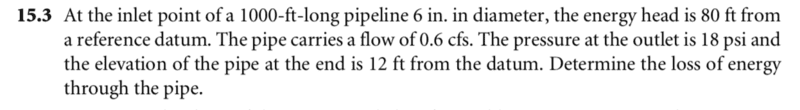# Hydraulics / fluid dynamics

• fayan77
Please explain.In summary, the conversation discusses using Bernoulli's equation to solve for pressure at P1 in a problem involving a pipe with varying pressure and height. The attempt at a solution involves using Bernoulli's equation to find P1, but the solution does not make sense as it results in a negative pressure. The conversation also mentions using the energy equation and including head losses, but it is unclear how to incorporate this into the solution.f

## Homework Statement## Homework Equations

y1+P1/##\gamma##+##\alpha##v12/2g = y2+P2/##\gamma##+##\alpha##v22/2g + hL

## The Attempt at a Solution

so it gives me pressure at p2 but not p1. I tried using Bernoulli's equation to find p1 i did plugged into energy equation above and got an answer but it did not make sense i got hL = .01 ft (I assumed that v1 = v2 but i do not think that is valid)

#### Attachments

Please post your working (and not as an image - those are for textbook extracts and diagrams).

...That is the problem exercise. Do you want me to type it instead?
I would like some direction so I can try it and post my work. Am I correct to use Bernoulli's equation to find P1? and will velocities be the same in both sections?

That is the problem exercise. Do you want me to type it instead?
The image you posted is fine. I am asking you to post your own working, but not as an image. I find that if I do not say that up front the working gets posted as an image which is hard to read and even harder to comment on.
Am I correct to use Bernoulli's equation to find P1? and will velocities be the same in both sections?
Yes and yes.

•Delta2
ok, So I start off with Bernoulli's equation solving for P1. Because v1 = v2 the kinetic energy term will cancel out leaving us with

P1 + ##\rho##gh1 = P2 + ##\rho##gh2
P1 +(1.94)(32.2)(80) = 2592 + (1.94)(32.3)(12)
P1 = -1655.8 psf
This does not make sense to me ( having a negative pressure at the top of the system)
Anyways now I will use the energy equation assume ##\alpha## = 1 also because v1 = v2 velocity head will cancel out from equation
y1 + P1/##\gamma## +##\alpha##v12/2g = y2 + P2/##\gamma## +##\alpha##v22/2g + hL
80 + (-1655.8/62.4) = 12 + (2592/62.4) + hL
hL = -.07 ft
This does not make sense to me (negative head) also I am certain that I am approaching this the wrong way because I did not use all the givens in the problem like Q, and L of pipe, and diameter of the pipe.

I start off with Bernoulli's equation
Ok, I misunderstood what you meant by that in post #1.
Bernoulli's equation is an energy equation, but it assumes no losses. I took your "relevant equation" to be a modified Bernoulli that allowed for losses. However, I am not familiar with this form, so please explain what α and γ represent, or post a link.
I would take the inlet pressure to be atmospheric and the outlet pressure to be 18 psi higher. I am not sure what the height info means, but it sounds like there is a drop of 68 ft along the pipe. That's a bit over two atmospheres, so with no flow one would expect a pressure difference of over 30 psi.
It is not clear what form of answer is required... is it the percentage loss or rate of loss?

Divide Bernoulli's equation by ##\gamma## and include head losses. Everything is in terms of head (length) ##\gamma## = ##\rho##g alpha is coefficient correction

Divide Bernoulli's equation by ##\gamma## and include head losses. Everything is in terms of head (length) ##\gamma## = ##\rho##g alpha is coefficient correction
Ok, that's about what I guessed. Does the "coefficient correction" represent the the loss due to drag? Or does hL represent energy loss?